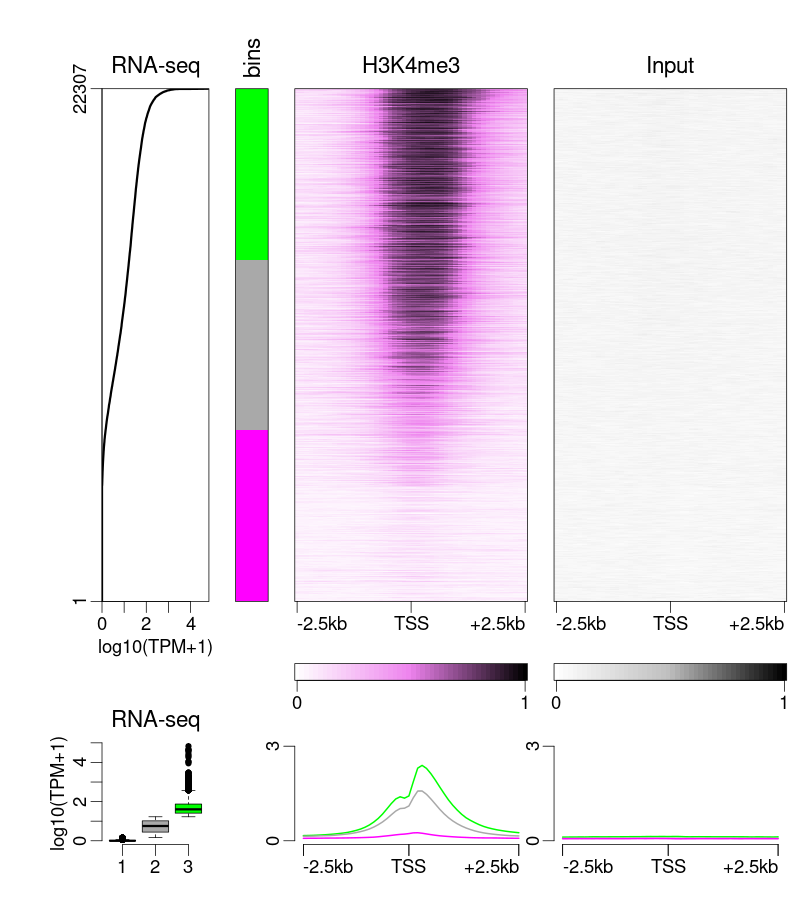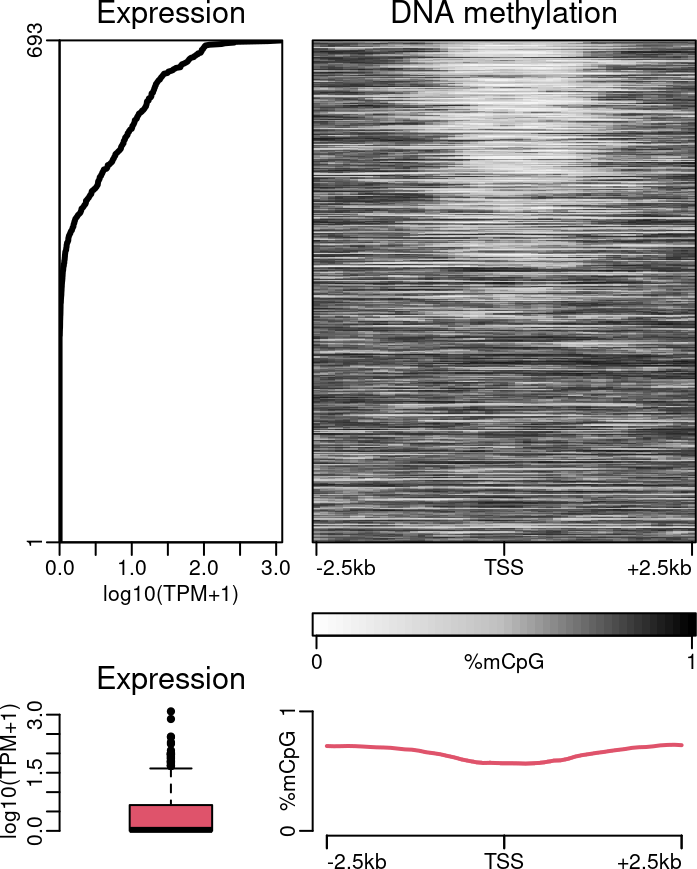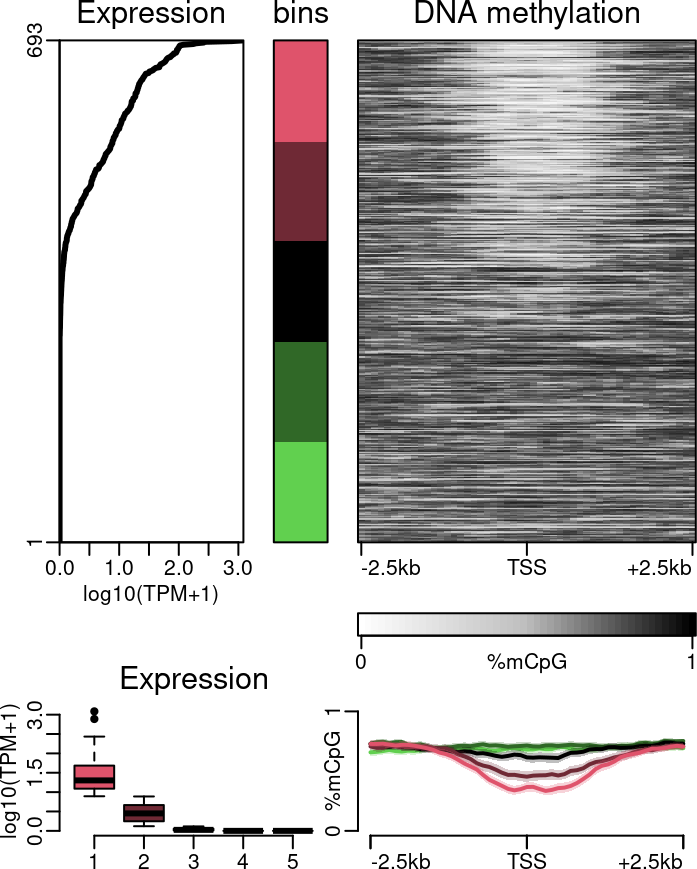# 1 IntroductionExample of epistack outputs

The `epistack` package main objective is the visualizations of stacks of genomic tracks (such as, but not restricted to, ChIP-seq or DNA methyation data) centered at genomic regions of interest. `epistack` needs three different inputs:

• a genomic score objects, such as ChIP-seq coverage or DNA methylation values, provided as a `GRanges` (easily obtained from `bigwig` or `bam` files)
• a list of feature of interest, such as peaks or transcription start sites, provided as a `GRanges` (easily obtained from `gtf` or `bed` files)
• a score to sort the features, such as peak height, or gene expression value

Each inputs are then combined in a single `RangedSummarizedExperiment` object use by epistack’s ploting functions.

After introducing epistack’s plotting capacity, this document will present two use cases:

• plotting ChIP-seq signal at peaks, to investigate peak quality
• plotting DNA methylation and ChIP-seq signals at gene promoters sorted according to gene expression, to investigate the associations between an epigenetic mark and gene expression

# 2 Epistack visualisation

Epistack is a visualisation package. It uses a `RangedSummarizedExperiment` object as input, with matrices embeded as assays. We will discuss how to build such input obects in the next section. For now on, we will focus on the visualisation functions using the example dataset included in the package.

The dataset can be accessed with:

``````library(GenomicRanges)
library(SummarizedExperiment)
library(epistack)

data("stackepi")
stackepi
#> class: RangedSummarizedExperiment
#> dim: 693 51
#> assays(1): DNAme
#> rownames(693): ENSSSCG00000016737 ENSSSCG00000036350 ...
#>   ENSSSCG00000024209 ENSSSCG00000048227
#> rowData names(3): gene_id exp score
#> colnames(51): window_1 window_2 ... window_50 window_51
#> colData names(0):``````

## 2.1 The `plotEpisatck()` function

This dataset can be visualised with the `plotEpistack()` function. The first parameter is the input `RangedSummarizedExperiment` object.

The second (optionnal) parameter, `assays` specifies which assay(s) should be displayed as heatmap(s). In the `stackepi` dataset, only one track is present, with an assay names `DNAme`. Note that it is possible to have several different tracks embeded in the same `RangedSummarizedExperiment` object, as demonstarted in the next sections.

An aditional `metric_col` is used, to display score associated with each anchor region, such as expression values or peak scores. Optionaly, the `metric_col` can be transformed before ploting using the `metric_transfunc` parameters.

``````plotEpistack(
stackepi,
assay = "DNAme", metric_col = "exp",
ylim = c(0, 1), zlim = c(0, 1),
x_labels = c("-2.5kb", "TSS", "+2.5kb"),
titles = "DNA methylation", legends = "%mCpG",
metric_title = "Expression", metric_label = "log10(TPM+1)",
metric_transfunc = function(x) log10(x+1)
)``````If a `bin` column is present, it is used to generate one average profile per bin.

``````stackepi <- addBins(stackepi, nbins = 5)

plotEpistack(
stackepi,
assay = "DNAme", metric_col = "exp",
ylim = c(0, 1), zlim = c(0, 1),
x_labels = c("-2.5kb", "TSS", "+2.5kb"),
titles = "DNA methylation", legends = "%mCpG",
metric_title = "Expression", metric_label = "log10(TPM+1)",
metric_transfunc = function(x) log10(x+1)
)``````Colours can be changed using dedicated parameters:

``````plotEpistack(
stackepi,
assay = "DNAme", metric_col = "exp",
ylim = c(0, 1), zlim = c(0, 1),
x_labels = c("-2.5kb", "TSS", "+2.5kb"),
titles = "DNA methylation", legends = "%mCpG",
metric_title = "Expression", metric_label = "log10(TPM+1)",
metric_transfunc = function(x) log10(x+1),
tints = "dodgerblue",
bin_palette = rainbow
)``````